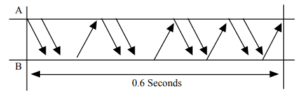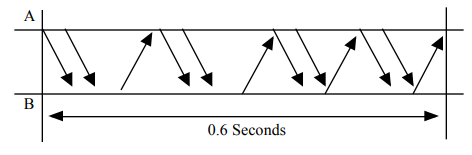# Ethernet in Networking | Practice Problems

## Ethernet in Networking-

Before you go through this article, make sure that you have gone through the previous article on Ethernet.

We have discussed-

• Ethernet is one of the standard LAN technologies used to build wired LANs.
• Ethernet uses bus topology in which all the stations are connected to a half duplex link.
• Ethernet uses CSMA / CD as an access control method.

## Problem-01:

Which of the following characteristic is most basic to LAN?

1. Bit rate
2. Delay x Bandwidth Product
3. Geographical distance
4. Cost

## Solution-

• Geographical distance is the basic criteria on which networks are classified.
• On the basis of geographical distance, networks are classified as LAN, MAN, WAN.
• Thus,Option (C) is correct.

## Problem-02:

On an Ethernet LAN when a collision is detected, the sending station-

1. continues to send the transmission
2. temporarily quits the transmission
3. notifies the destination of an error
4. permanently quits the transmission

## Solution-

• Ethernet uses CSMA / CD as access control method.
• On detecting a collision, the sending station temporarily quits the transmission.
• Transmitting station waits for Back Off time and then tries again.
• Thus, Option (B) is correct.

## Problem-03:

Ethernet implements _________ service for its operation.

1. connection oriented
2. connection less
3. Both A and B
4. Either A or B

## Solution-

### REMEMBER

• Connection oriented service involves allocation of the dedicated resources.
• Connection less service does not involve allocation of dedicated resources.
• TCP and Virtual Circuits are connection oriented services.
• IP, Ethernet and Token Ring are connection less services.
• Datagrams are connection less, that is why IP is connection less.

When an Ethernet frame is sent,

• Destination is never expected to reserve the buffer or any other resource for the incoming frame.
• The data is simply dumped at the destination side.
• So, it is connectionless.
• Thus, Option (B) is correct.

## Problem-04:

The collision domain of Fast Ethernet is limited to ______ meters.

1. 2.5
2. 25
3. 250
4. 2500

## Solution-

• Collision domain defines the number of stations that can get involved in the collision when connected to a LAN.
• In the given question, collision domain refers to maximum distance a LAN can run to detect the collisions.
• Ethernet uses CSMA / CD as access control method.

In CSMA / CD, condition to detect collisions is-

Distance <= (Length x speed) / (2 x bandwidth)

On substituting the values, we get the value of distance.

### REMEMBER

• For normal Ethernet, collision domain = 2500 meters.
• For Fast Ethernet, collision domain = 250 meters.
• For Gigabit Ethernet, collision domain = 25 meters.

Thus, Option (C) is correct.

## Problem-05:

The efficiency of Ethernet-

1. increases when propagation delay and transmission delay are low
2. increases when propagation delay and transmission delay are high
3. increases when propagation delay is low and transmission delay is high
4. increases when propagation delay is high and transmission delay is low

## Solution-

• Efficiency of Ethernet = 1 / ( 1 + 6.44a) where a = Tp / Tt.
• Thus, Option (C) is correct.

## Problem-06:

What is the baud rate of the standard 10 Mbps 802.3 LAN?

1. 20 mega baud
2. 10 mega baud
3. 25 mega baud
4. 40 mega baud

## Solution-

LAN uses Manchester Encoding Technique where-

Baud rate = 2 x Bit rate

For 10 Mbps,

Baud rate

= 2 x 10 mega baud

= 20 mega baud

Thus, Option (A) is correct.

## Problem-07:

Consider a 10 Mbps Ethernet LAN that has stations attached to a 2.5 km long coaxial cable. Given that the transmission speed is 2.3 x 108 m/sec, the packet size is 128 bytes out of which 30 bytes are overhead, find the effective transmission rate and maximum rate at which the network can send data.

## Solution-

Given-

• Bandwidth = 10 Mbps
• Distance = 2.5 km
• Transmission speed = 2.3 x 108 m/sec
• Total packet size = 128 bytes

### Calculating Transmission Delay-

Transmission delay (Tt)

= Packet size / Bandwidth

= 128 bytes / 10 Mbps

= (128 x 8 bits) / (10 x 106 bits per sec)

= 1024 / 107 sec

= 102.4 μsec

### Calculating Propagation Delay-

Propagation delay (Tp)

= Distance / Speed

= 2.5 km / (2.3 x 108 m/sec)

= (2.5 x 103 m) / (2.3 x 108 m/sec)

= 1.08 x 10-5 sec

= 10.8 μsec

### Calculating Value of ‘a’-

a

= Tp / Tt

= 10.8 μsec / 102.4 μsec

= 0.105

### Calculating Efficiency-

Efficiency(η)

= 1 / (1 + 6.44 x a)

= 1 / (1 + 6.44 x 0.105)

= 1 / 1.67

= 0.59

= 59%

### Calculating Maximum Rate-

Maximum rate or Throughput

= Efficiency x Bandwidth

= 0.59 x 10 Mbps

= 5.9 Mbps

### Calculating Effective Transmission Rate-

Effective transmission rate

= Throughput x (128-30 / 128)

= 5.9 Mbps x (98 / 128)

= 0.77 x 5.9 Mbps

= 4.52 Mbps

## Problem-08:

The following frame transition diagram shows an exchange of Ethernet frames between two computers, A and B connected via a 10BT Hub. Each frame sent by computer A contains 1500 B of Ethernet payload data, while each frame sent by computer B contains 40 B of Ethernet payload data. Calculate the average utilization of the media during this exchange.1. 10%
2. 1.7%
3. 20%
4. 15.2%

## Solution-

### Calculating Data Sent By Computer A in One Frame-

Given-

• Each frame sent by computer A contains 1500 B of Ethernet payload data.
• This is divided as: 20 bytes of IP Header + 20 bytes of TCP Header + 1460 bytes of data.

So, Total bytes sent by computer A in one frame

= Preamble + SFD + Ethernet Header + Ethernet Payload + CRC

= 7 bytes + 1 byte + 14 bytes + 1500 bytes + 4 bytes

= 1526 bytes

### Calculating Data Sent By Computer A in 0.6 Seconds:

Computer A sends 8 frames in 0.6 seconds.

So, Total bytes sent by computer A in 0.6 seconds

= 8 x 1526 bytes

= 12208 bytes

### Calculating Data Sent By Computer B in One Frame-

Given-

• Each frame sent by computer B contains 40 B of Ethernet payload data.
• This is divided as: 20 bytes of IP Header + 20 bytes of TCP Header + 0 bytes of data.
• Since minimum data in the payload field of Ethernet must 46 bytes. So, extra 6 bytes are padded.

So, Total bytes sent by computer B in one frame

= Preamble + SFD + Ethernet Header + Ethernet Payload + CRC

= 7 bytes + 1 byte + 14 bytes + (40 bytes + 6 bytes) + 4 bytes

= 72 bytes

### Calculating Data Sent By Computer B in 0.6 Seconds-

Computer B sends 4 frames in 0.6 seconds.

So, Total bytes sent by computer B in 0.6 seconds

= 4 x 72 bytes

= 288 bytes

### Calculating Total Data Sent in 0.6 Seconds:

Total data flow that takes place in 0.6 seconds

= Total data sent by computer A in 0.6 seconds + Total data sent by computer B in 0.6 seconds

= 12208 bytes + 288 bytes

= 12496 bytes

= 99968 bits

### Calculating Throughput-

Throughput

= Amount of data that flows per second

= 99968 bits / 0.6 seconds

= 166613.33 bits/sec

### Calculating Utilization-

Throughput = Efficiency x Bandwidth

So, Efficiency or Utilization

= Throughput / Bandwidth

= (166613.33 bits per sec) / 10 Mbps

= 0.017

= 1.7%

Thus, Option (B) is correct.

## Problem-09:

3. Frames if it has been placed in promiscuous mode
4. All of the above

## Solution-

In a bus topology Ethernet,

• Ethernet Adaptor enables a computer to access an Ethernet Network.
• If one station sends a frame to other station, then other stations & Ethernet Adaptor also receives that frame.
• But they accept only those frames which are destined for them.
• Network administrator may set the network in promiscuous mode.
• This is done to monitor the activities going on in the network.
• So, if Ethernet Adaptor is set in promiscuous mode, it receives and accepts all the frames.
• Thus, Option (D) is correct.

Next Article- Types of Switching | Circuit Switching

Get more notes and other study material of Computer Networks.

Watch video lectures by visiting our YouTube channel LearnVidFun.

SummaryArticle Name
Ethernet in Networking | Practice Problems
Description
Practice Problems based on Ethernet. Ethernet in networking is a LAN technology. Ethernet frame format has several fields. Ethernet frame size range = [64 bytes, 1518 bytes]. Ethernet Header Size = 14 bytes.
Author
Publisher Name
Gate Vidyalay
Publisher Logo